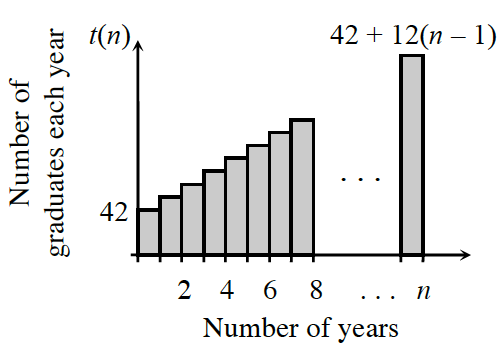### Home > CCA2 > Chapter Ch10 > Lesson 10.2.1 > Problem10-88

10-88.According to the school secretary, the size of the graduating class of Gauss High has steadily increased since the school has opened. She claims that the number of graduates each year forms the sequence $t(n) = 42 + 12(n - 1)$, where n is the number of years the high school has had students graduate. At the end of the year, the administration mails out invitations to all previous graduates to attend the upcoming graduation ceremony.

1. In this situation, what does a sequence represent? What does a series represent?

Remember that a sequence is a set of discrete numbers while a series is the sum of those numbers.

2. After the $10$th graduation ceremony, how many total graduates should there be? Explain how you found your answer.

Use the summation formula $S_{10}=\frac{10}{2}\left(t\left(1\right) + t\left(10\right)\right)$

3.The total number of Gauss High graduates after $n$ years can be represented by the graph below. If the alumni relations committee wants to invite all graduates to a party to celebrate the $n$th anniversary of the school, how may invitations will they need to send?

See part (b).